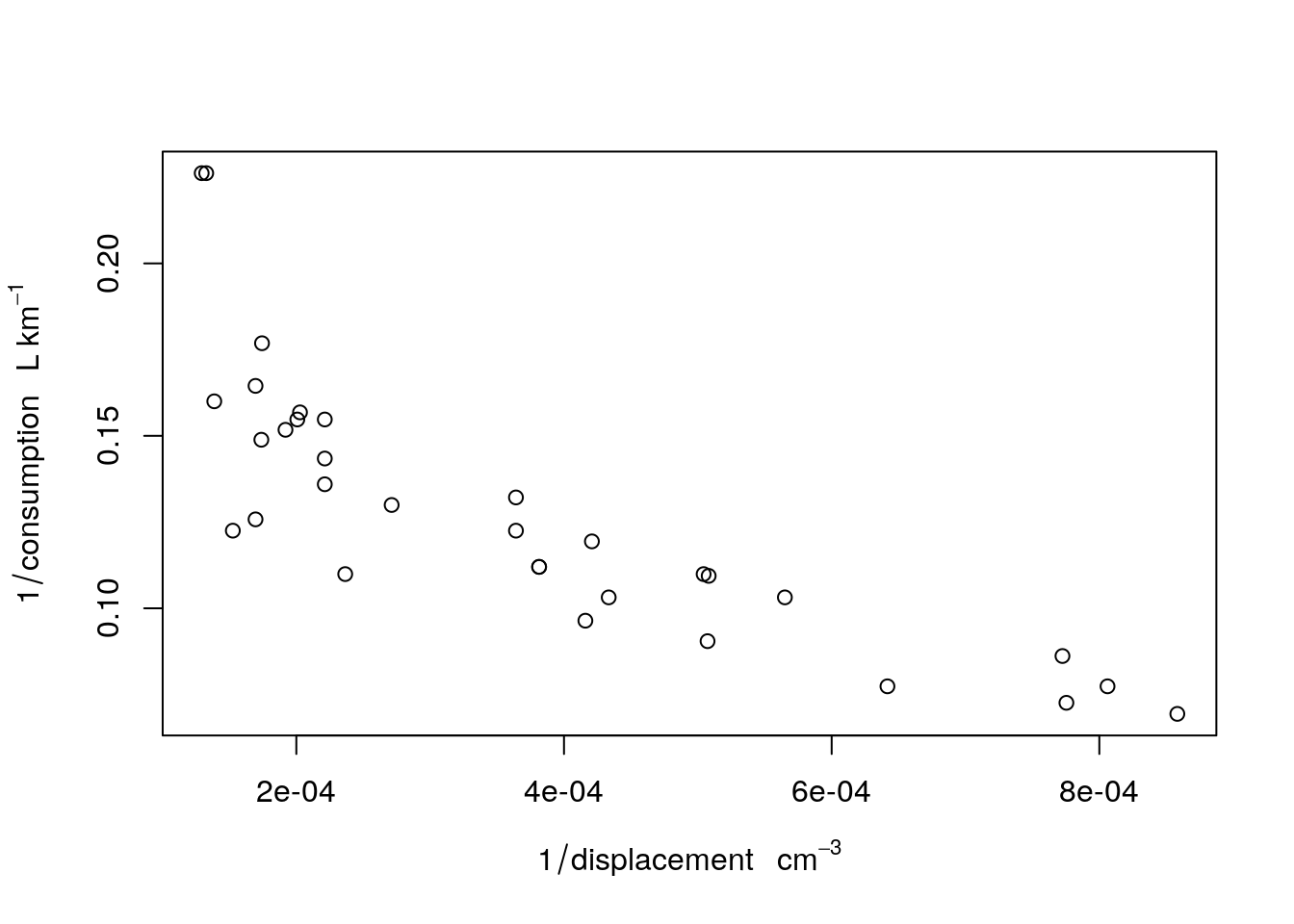R has little support for physical measurement units. The exception is formed by time differences: time differences objects of class difftime have a units attribute that can be modified:

t1 = Sys.time()
t2 = t1 + 3600
d = t2 - t1
class(d)
##  "difftime"
units(d)
##  "hours"
d
## Time difference of 1 hours
units(d) = "secs"
d
## Time difference of 3600 secs

We see here that the units method is used to retrieve and modify the unit of time differences.

The units package generalizes this idea to other physical units, building upon the udunits2 R package, which in turn is build upon the udunits2 C library. The udunits2 library provides the following operations:

• validating whether an expression, such as m/s is a valid physical unit
• verifying whether two units such as m/s and km/h are convertible
• converting values between two convertible units
• providing names and symbols for specific units
• handle different character encodings (utf8, ascii, iso-8859-1 and latin1)

The units R package uses R package udunits2 to extend R with functionality for manipulating numeric vectors that have physical measurement units associated with them, in a similar way as difftime objects behave.

## Setting units, unit conversion

Existing units are resolved from a database in the units package, called ud_units. We can see the first three elements of it by

library(units)
ud_units[1:3]
## $m ## 1 [m] ## ##$kg
## 1 [kg]
##
## $s ## 1 [s] We can set units to numerical values by set_units: (a <- set_units(runif(10), m/s)) ## Units: [m/s] ##  0.4518294 0.3910316 0.5732944 0.6965670 0.2450122 0.4020448 0.4025526 ##  0.7891220 0.5043406 0.1370050 the result, e.g. set_units(10, m/s) ## 10 [m/s] literally means "10 times 1 m divided by 1 s". In writing, the "1" values are omitted, and the multiplication is implicit. The units package comes with a list of over 3000 predefined units, length(ud_units) ##  3248 We can retrieve a single unit from the ud_units database by with(ud_units, km/h) ## 1 [km/h] ### Unit conversion When conversion is meaningful, such as hours to seconds or meters to kilometers, conversion can be done explicitly by setting the units of a vector b = a units(b) <- with(ud_units, km/h) b ## Units: [km/h] ##  1.6265858 1.4077138 2.0638598 2.5076413 0.8820439 1.4473614 1.4491894 ##  2.8408390 1.8156263 0.4932182 ## Basic manipulations ### Arithmetic operations Arithmetic operations verify units, and create new ones a + a ## Units: [m/s] ##  0.9036588 0.7820632 1.1465888 1.3931341 0.4900244 0.8040897 0.8051052 ##  1.5782439 1.0086813 0.2740101 a * a ## Units: [m^2/s^2] ##  0.20414981 0.15290573 0.32866646 0.48520562 0.06003097 0.16164006 ##  0.16204860 0.62271346 0.25435948 0.01877038 a ^ 2 ## Units: [m^2/s^2] ##  0.20414981 0.15290573 0.32866646 0.48520562 0.06003097 0.16164006 ##  0.16204860 0.62271346 0.25435948 0.01877038 a ** -2 ## Units: [s^2/m^2] ##  4.898364 6.539977 3.042598 2.060982 16.658067 6.186585 6.170988 ##  1.605875 3.931444 53.275420 and convert to the units of the first argument if necessary: a + b # m/s + km/h -> m/s ## Units: [m/s] ##  0.9036588 0.7820632 1.1465888 1.3931341 0.4900244 0.8040897 0.8051052 ##  1.5782439 1.0086813 0.2740101 Currently, powers are only supported for integer powers, so using a ** 2.5 would result in an error. ### Unit simplification There are some basic simplification of units: t <- with(ud_units, s) a * t ## Units: [m] ##  0.4518294 0.3910316 0.5732944 0.6965670 0.2450122 0.4020448 0.4025526 ##  0.7891220 0.5043406 0.1370050 which also work when units need to be converted before they can be simplified: t <- with(ud_units, min) a * t ## Units: [m] ##  27.109764 23.461897 34.397663 41.794022 14.700732 24.122691 24.153157 ##  47.347317 30.260438 8.220303 Simplification to unit-less values gives the "1" as unit: m <- with(ud_units, m) a * t / m ## Units:  ##  27.109764 23.461897 34.397663 41.794022 14.700732 24.122691 24.153157 ##  47.347317 30.260438 8.220303 Allowed operations that require convertible units are +, -, ==, !=, <, >, <=, >=. Operations that lead to new units are *, /, and the power operations ** and ^. ### Mathematical functions Mathematical operations allowed are: abs, sign, floor, ceiling, trunc, round, signif, log, cumsum, cummax, cummin. signif(a ** 2 / 3, 3) ## Units: [m^2/s^2] ##  0.06800 0.05100 0.11000 0.16200 0.02000 0.05390 0.05400 0.20800 0.08480 ##  0.00626 cumsum(a) ## Units: [m/s] ##  0.4518294 0.8428610 1.4161554 2.1127224 2.3577346 2.7597795 3.1623321 ##  3.9514540 4.4557947 4.5927997 log(a) # base defaults to exp(1) ## Units: [(ln(re 1 m.s-1))] ##  -0.7944506 -0.9389668 -0.5563559 -0.3615913 -1.4064473 -0.9111916 ##  -0.9099295 -0.2368344 -0.6845034 -1.9877375 log(a, base = 10) ## Units: [(lg(re 1 m.s-1))] ##  -0.3450255 -0.4077881 -0.2416223 -0.1570371 -0.6108123 -0.3957255 ##  -0.3951774 -0.1028559 -0.2972760 -0.8632634 log(a, base = 2) ## Units: [(lb(re 1 m.s-1))] ##  -1.1461499 -1.3546428 -0.8026519 -0.5216659 -2.0290745 -1.3145717 ##  -1.3127507 -0.3416798 -0.9875296 -2.8676991 ### Summary functions Summary functions sum, min, max, and range are allowed: sum(a) ## 4.5928 [m/s] min(a) ## 0.137005 [m/s] max(a) ## 0.789122 [m/s] range(a) ## Units: [m/s] ##  0.137005 0.789122 with(ud_units, min(m/s, km/h)) # converts to first unit: ## 0.2777778 [m/s] ### Printing Following difftime, printing behaves differently for length-one vectors: a ## Units: [m/s] ##  0.4518294 0.3910316 0.5732944 0.6965670 0.2450122 0.4020448 0.4025526 ##  0.7891220 0.5043406 0.1370050 a ## 0.4518294 [m/s] ### Subsetting The usual subsetting rules work: a[2:5] ## Units: [m/s] ##  0.3910316 0.5732944 0.6965670 0.2450122 a[-(1:9)] ## 0.137005 [m/s] ### Concatenation c(a,a) ## Units: [m/s] ##  0.4518294 0.3910316 0.5732944 0.6965670 0.2450122 0.4020448 0.4025526 ##  0.7891220 0.5043406 0.1370050 0.4518294 0.3910316 0.5732944 0.6965670 ##  0.2450122 0.4020448 0.4025526 0.7891220 0.5043406 0.1370050 concatenation converts to the units of the first argument, if necessary: c(a,b) # m/s, km/h -> m/s ## Units: [m/s] ##  0.4518294 0.3910316 0.5732944 0.6965670 0.2450122 0.4020448 0.4025526 ##  0.7891220 0.5043406 0.1370050 0.4518294 0.3910316 0.5732944 0.6965670 ##  0.2450122 0.4020448 0.4025526 0.7891220 0.5043406 0.1370050 c(b,a) # km/h, m/s -> km/h ## Units: [km/h] ##  1.6265858 1.4077138 2.0638598 2.5076413 0.8820439 1.4473614 1.4491894 ##  2.8408390 1.8156263 0.4932182 1.6265858 1.4077138 2.0638598 2.5076413 ##  0.8820439 1.4473614 1.4491894 2.8408390 1.8156263 0.4932182 ## Conversion to/from difftime From difftime to units: t1 = Sys.time() t2 = t1 + 3600 d = t2 - t1 (du = as_units(d)) ## 1 [h] vice versa: (dt = as_difftime(du)) ## Time difference of 1 hours class(dt) ##  "difftime" ## units in matrix objects set_units(matrix(1:4,2,2), m/s) ## Units: [m/s] ## [,1] [,2] ## [1,] 1 3 ## [2,] 2 4 set_units(matrix(1:4,2,2), m/s * m/s) ## Units: [m^2/s^2] ## [,1] [,2] ## [1,] 1 3 ## [2,] 2 4 but set_units(matrix(1:4,2,2), m/s) %*% set_units(4:3, m/s) ## [,1] ## [1,] 13 ## [2,] 20 strips units. ## units objects in data.frames units in data.frame objects are printed, but do not appear in summary:. set.seed(131) d <- data.frame(x = runif(4), y = set_units(runif(4), s), z = set_units(1:4, m/s)) d ## x y z ## 1 0.2064370 0.8463468 [s] 1 [m/s] ## 2 0.1249422 0.5292048 [s] 2 [m/s] ## 3 0.2932732 0.5186254 [s] 3 [m/s] ## 4 0.3757797 0.2378545 [s] 4 [m/s] summary(d) ## x y z ## Min. :0.1249 Min. :0.2379 Min. :1.00 ## 1st Qu.:0.1861 1st Qu.:0.4484 1st Qu.:1.75 ## Median :0.2499 Median :0.5239 Median :2.50 ## Mean :0.2501 Mean :0.5330 Mean :2.50 ## 3rd Qu.:0.3139 3rd Qu.:0.6085 3rd Qu.:3.25 ## Max. :0.3758 Max. :0.8463 Max. :4.00 d$yz = with(d, y * z)
d
##           x             y       z            yz
## 1 0.2064370 0.8463468 [s] 1 [m/s] 0.8463468 [m]
## 2 0.1249422 0.5292048 [s] 2 [m/s] 1.0584095 [m]
## 3 0.2932732 0.5186254 [s] 3 [m/s] 1.5558761 [m]
## 4 0.3757797 0.2378545 [s] 4 [m/s] 0.9514180 [m]
d[1, "yz"]
## 0.8463468 [m]

## formatting

Units are often written in the form m2 s-1, for square meter per second. This can be defined as unit, and also parsed by as_units:

(x = 1:10 * as_units("m2 s-1"))
## Units: [m^2/s]
##    1  2  3  4  5  6  7  8  9 10

udunits understands such string, and can convert them

y = 1:10 * with(ud_units, m^2/s)
x + y
## Units: [m^2/s]
##    2  4  6  8 10 12 14 16 18 20

Printing units in this form is done by

deparse_unit(x)
##  "m2 s-1"

## plotting

Base scatter plots and histograms support automatic unit placement in axis labels. In the following example we first convert to SI units. (Unit in needs a bit special treatment, because in is a reserved word in R.)

mar = par("mar") + c(0, .3, 0, 0)
displacement = mtcars$disp * ud_units[["in"]]^3 units(displacement) = with(ud_units, cm^3) weight = mtcars$wt * 1000 * with(ud_units, lb)
units(weight) = with(ud_units, kg)
par(mar = mar)
plot(weight, displacement)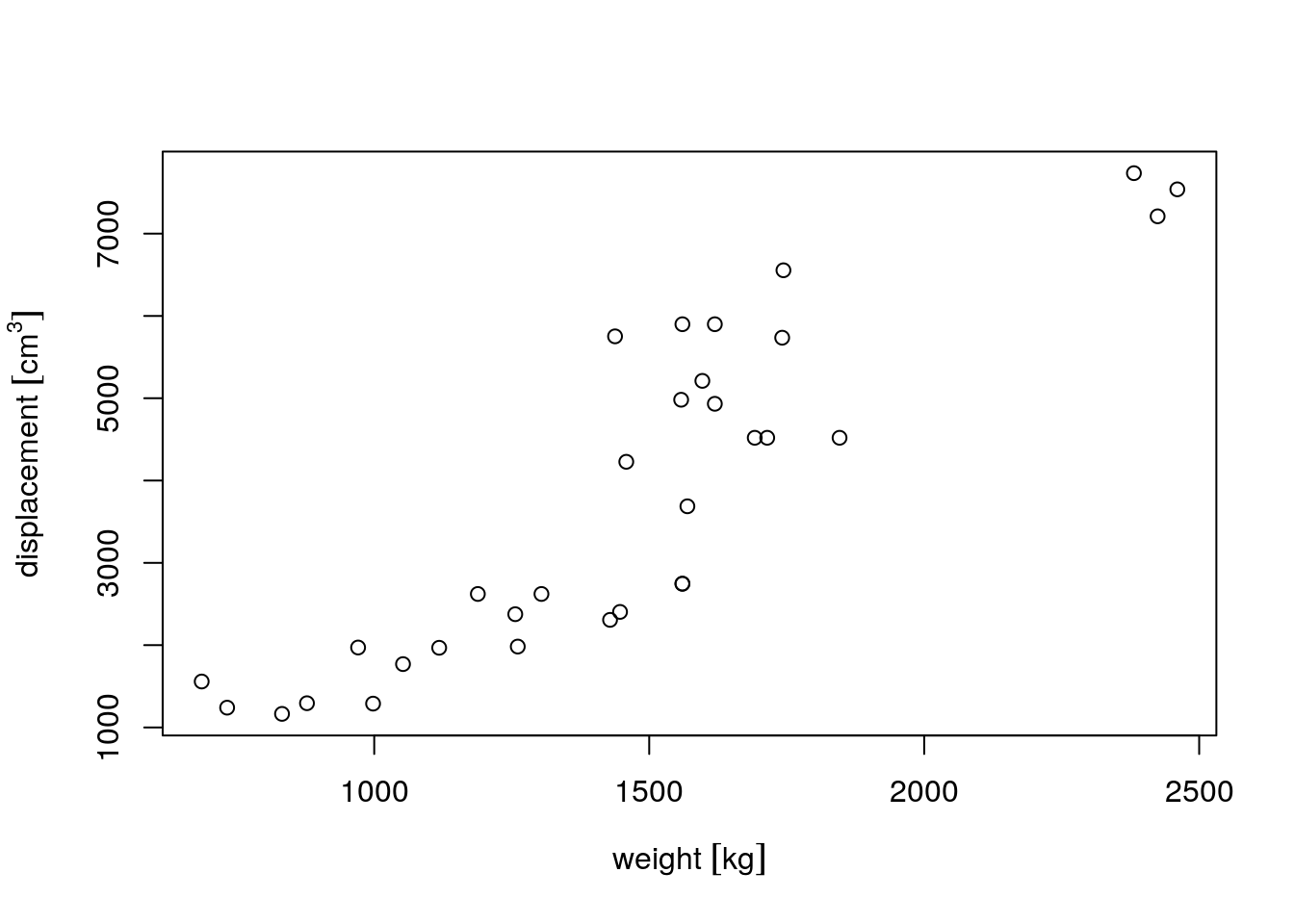We can change grouping symbols from [ ] into ( ):

units_options(group = c("(", ")") )  # parenthesis instead of square brackets
par(mar = mar)
plot(weight, displacement)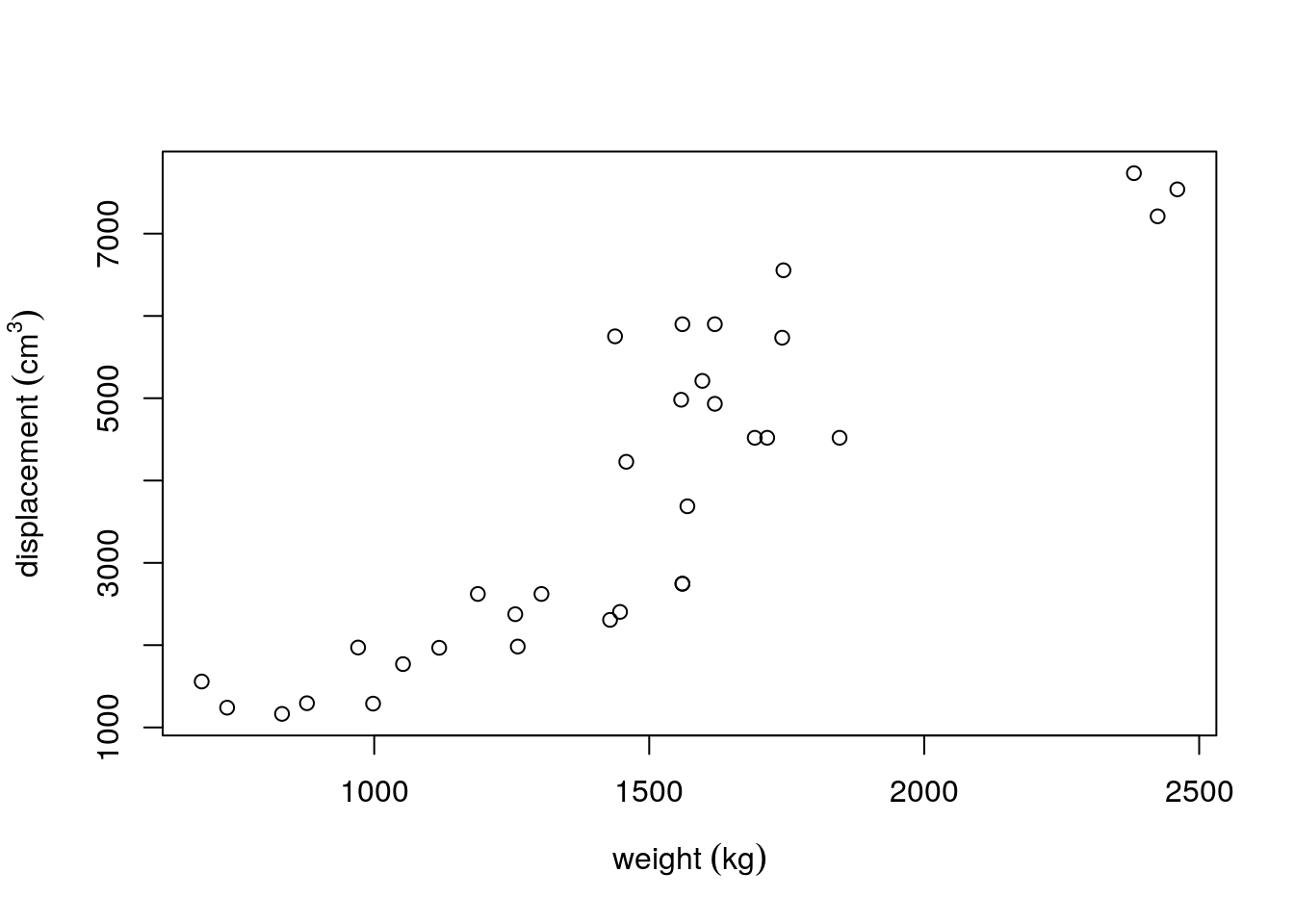We can also remove grouping symbols, increase space between variable name and unit by:

units_options(sep = c("~~~", "~"), group = c("", ""))  # no brackets; extra space
par(mar = mar)
plot(weight, displacement)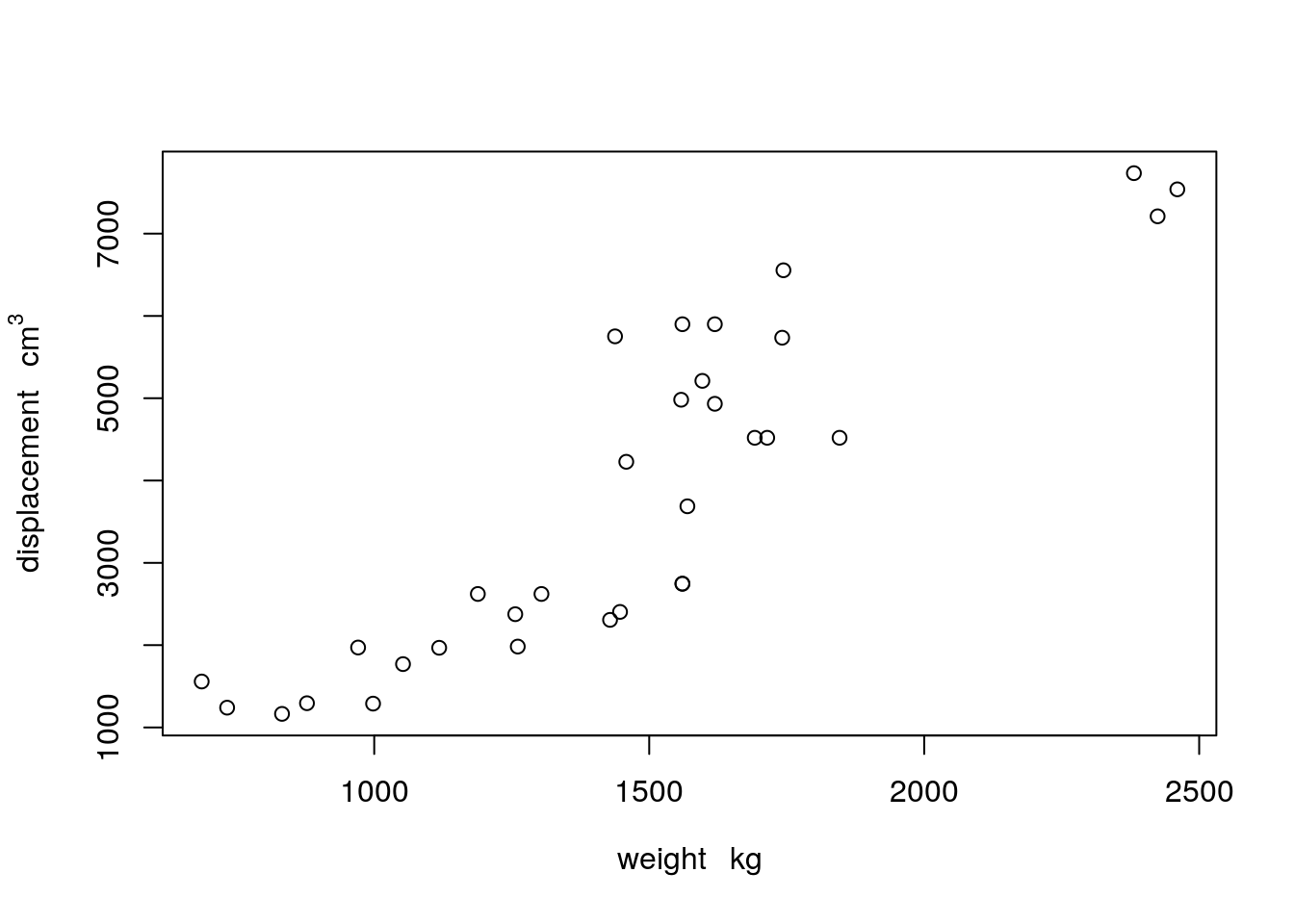More complex units can be plotted either with negative powers, or as divisions, by modifying one of units's global options using units_options:

gallon = as_units("gallon")
consumption = mtcars\$mpg * with(ud_units, mi/gallon)
units(consumption) = with(ud_units, km/l)
par(mar = mar)
plot(displacement, consumption) # division in consumption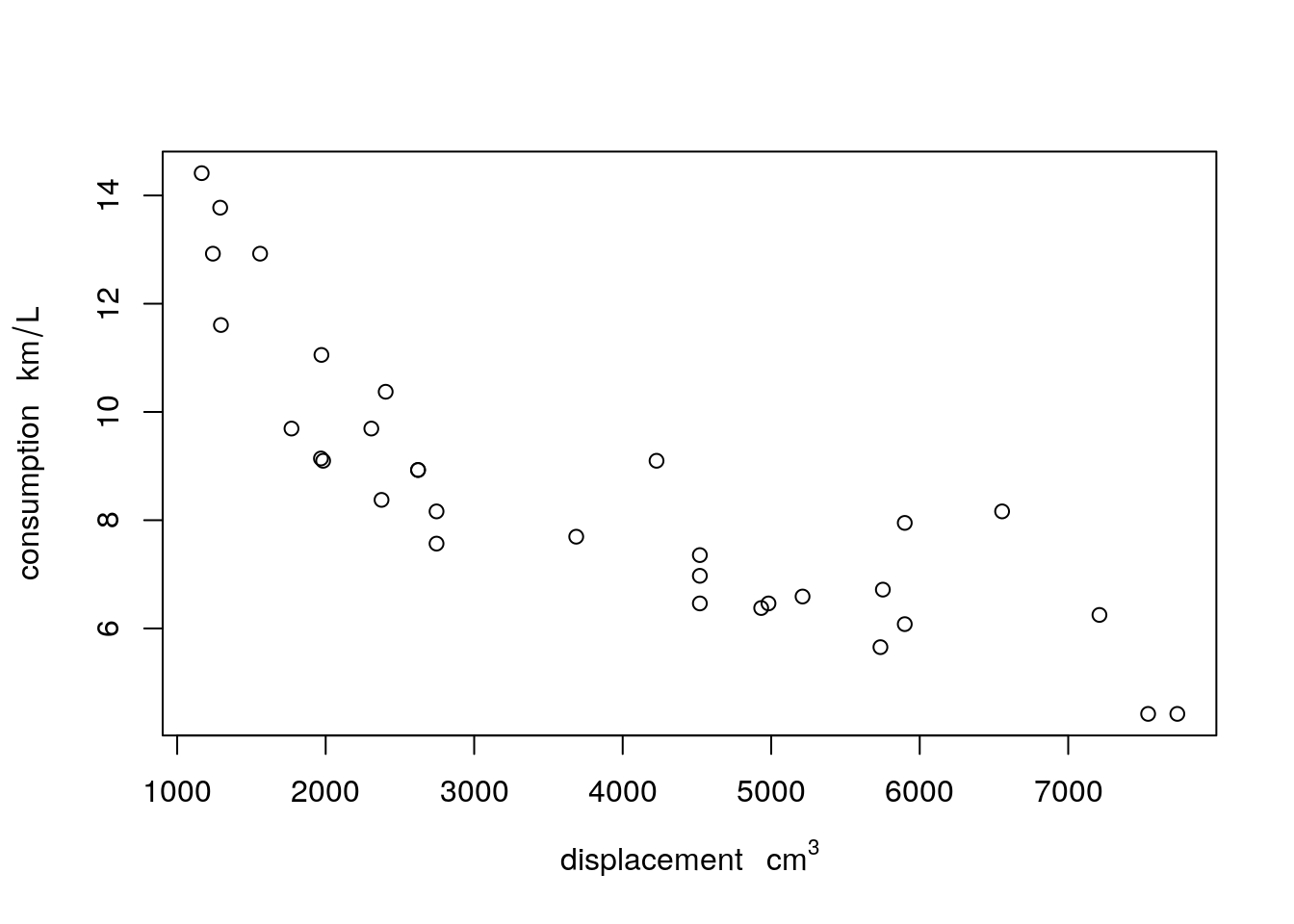units_options(negative_power = TRUE) # division becomes ^-1
plot(displacement, consumption) # division in consumption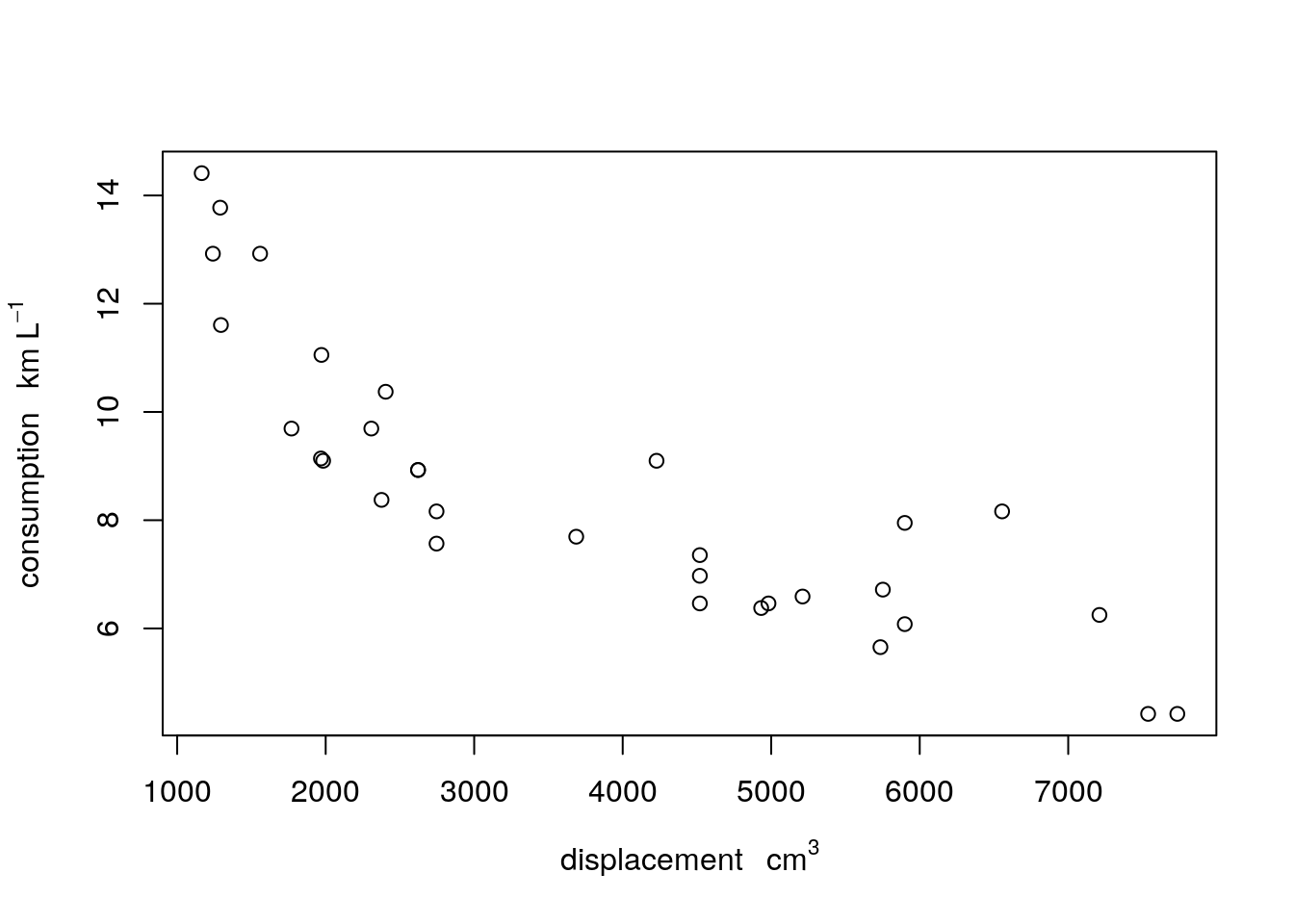As usual, units modify automatically in expressions:

units_options(negative_power = TRUE) # division becomes ^-1
par(mar = mar)
plot(displacement, consumption)plot(1/displacement, 1/consumption)